# 数字信号处理中卷积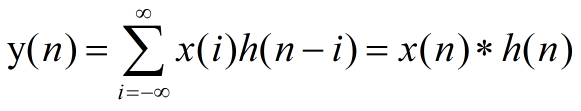# 数字图像处理中卷积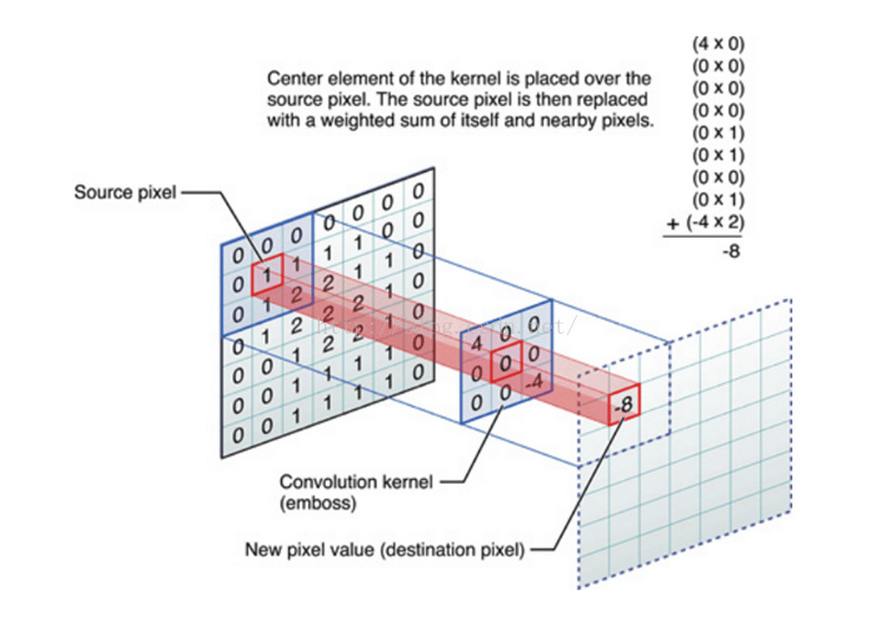1）卷积核的大小一般是奇数，这样的话它是按照中间的像素点中心对称的，所以卷积核一般都是3x3，5x5或者7x7。有中心了，也有了半径的称呼，例如5x5大小的核的半径就是2。
2）卷积核所有的元素之和一般要等于1，这是为了原始图像的能量（亮度）守恒。其实也有卷积核元素相加不为1的情况，下面就会说到。
3）如果滤波器矩阵所有元素之和大于1，那么滤波后的图像就会比原图像更亮，反之，如果小于1，那么得到的图像就会变暗。如果和为0，图像不会变黑，但也会非常暗。
4）对于滤波后的结构，可能会出现负数或者大于255的数值。对这种情况，我们将他们直接截断到0和255之间即可。对于负数，也可以取绝对值。

# 边界补充问题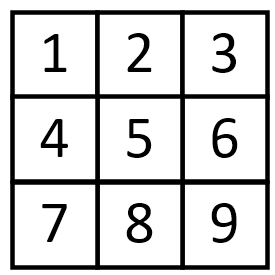## 补零填充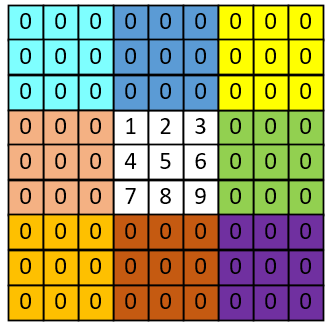## 边界复制填充## 镜像填充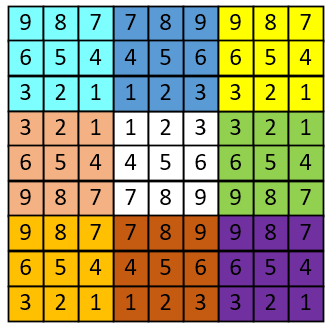## 块填充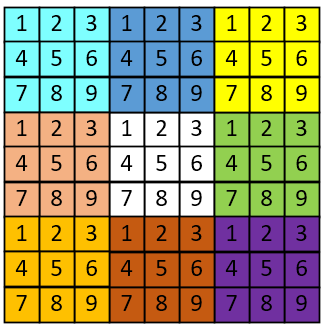# 不同卷积核下卷积意义

## 一个没有任何作用的卷积核：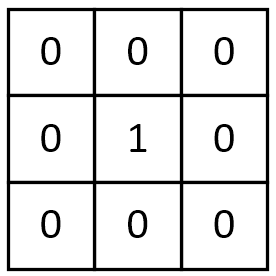## 平滑均值滤波：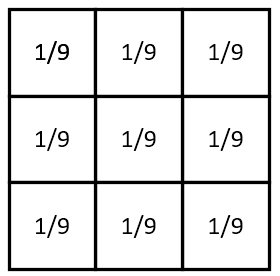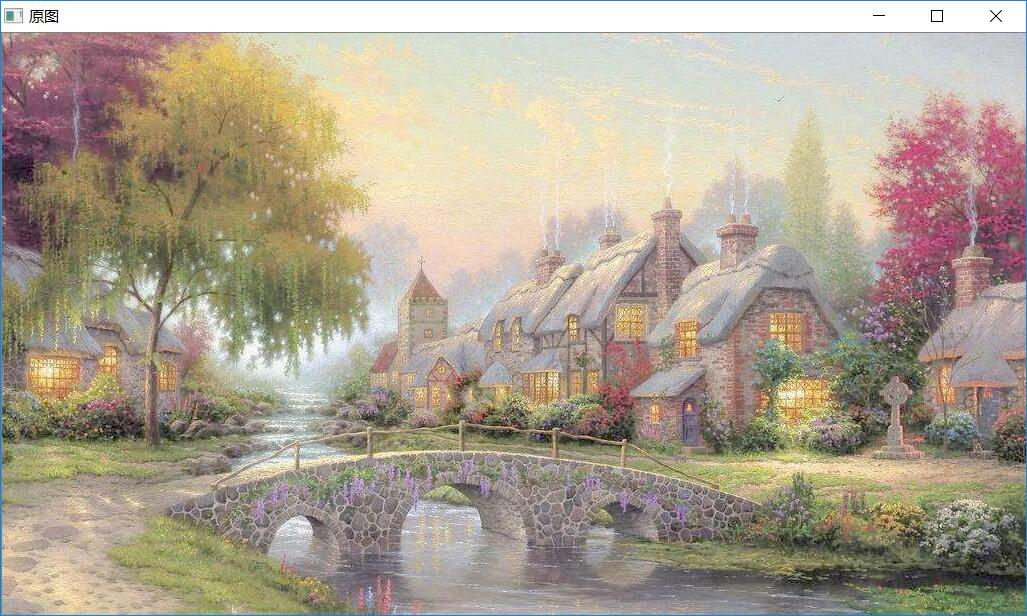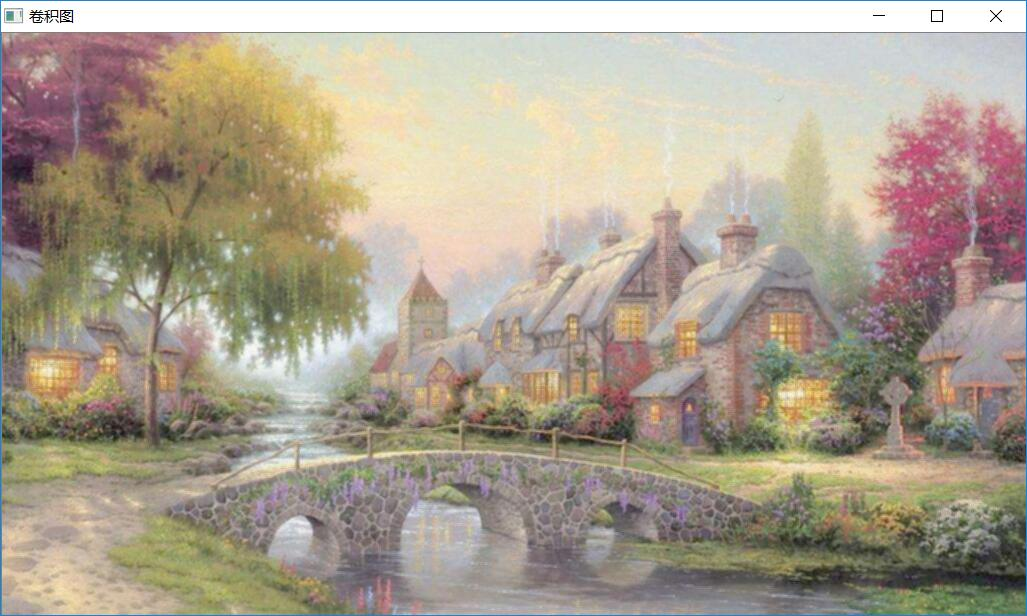## 高斯平滑：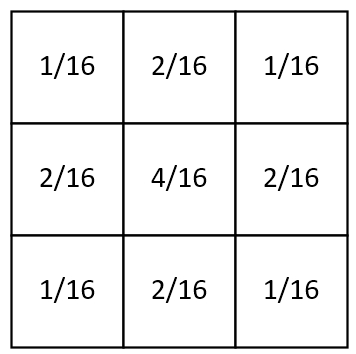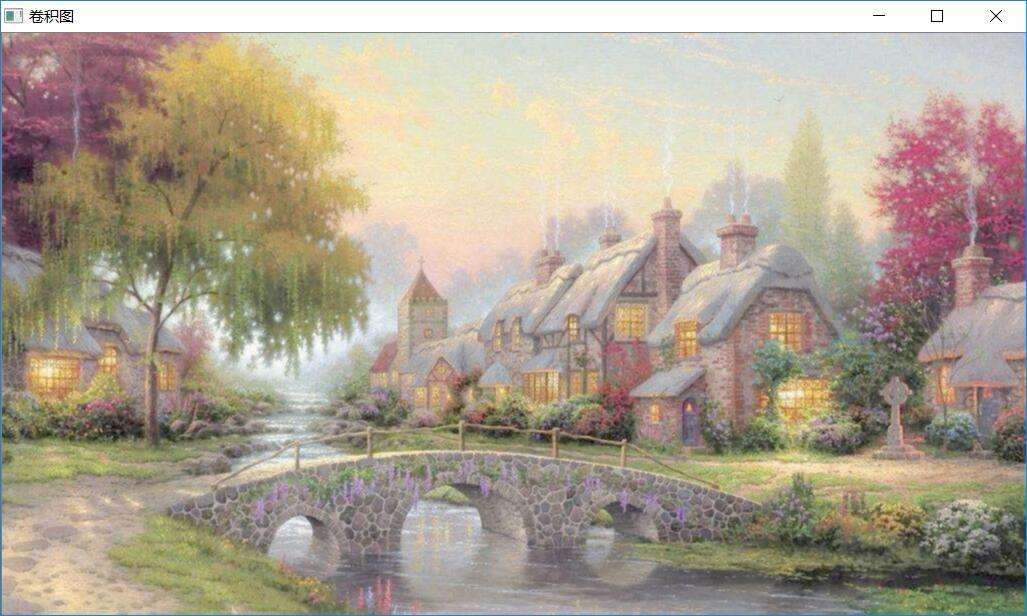## 图像锐化：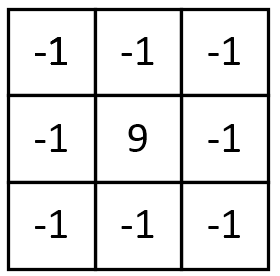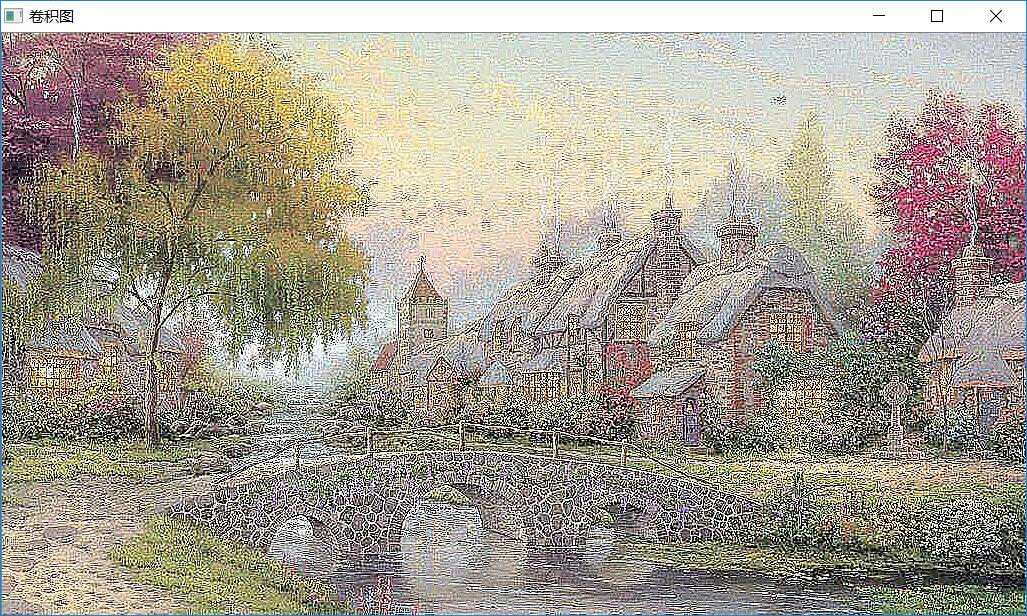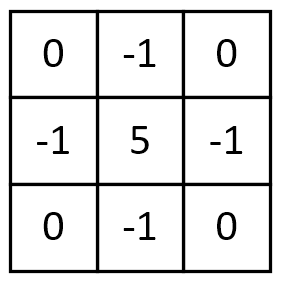## 梯度Prewitt：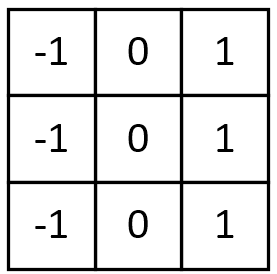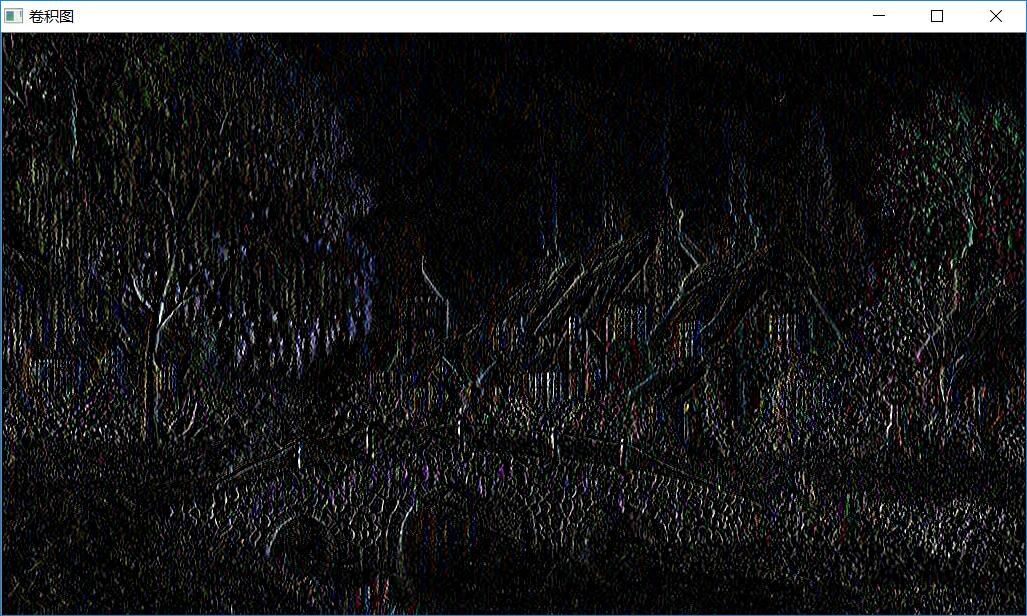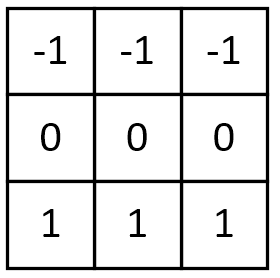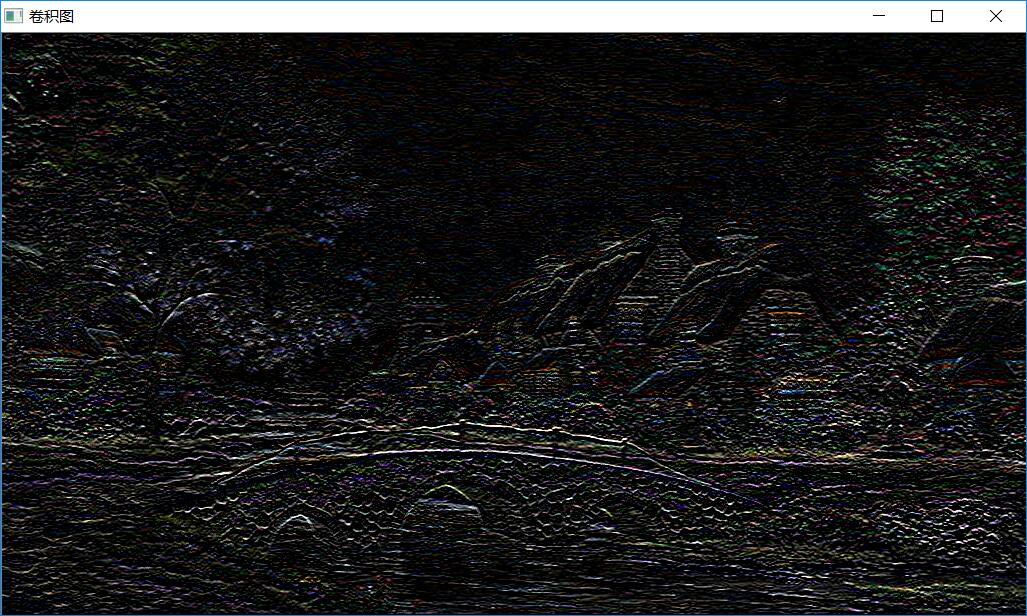## Soble边缘检测：

Soble与上述卷积核不同之处在于，Soble更强调了和边缘相邻的像素点对边缘的影响。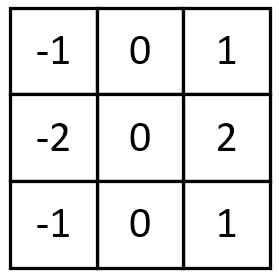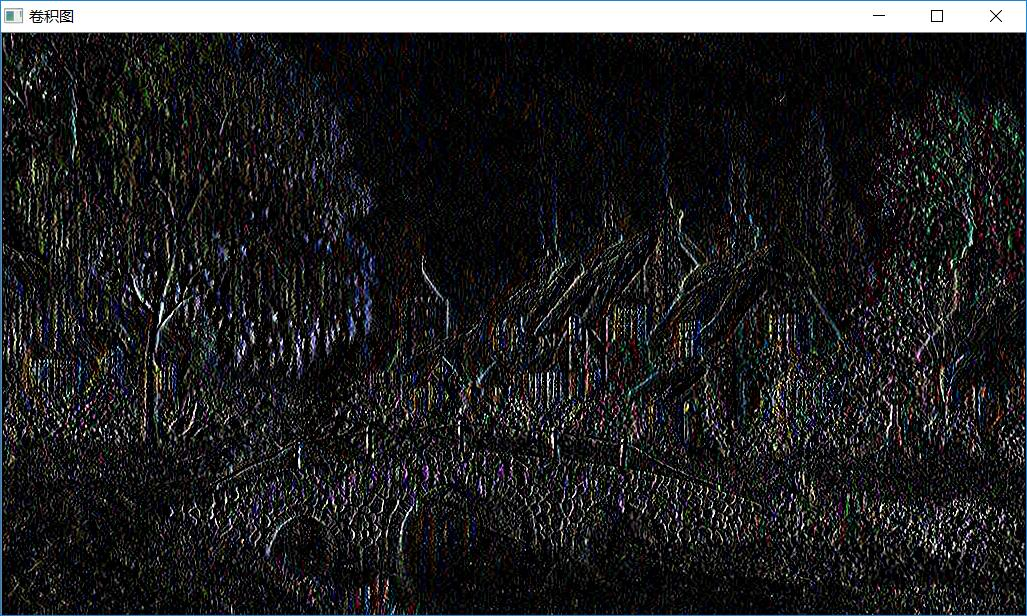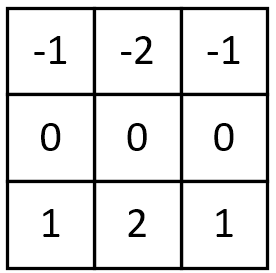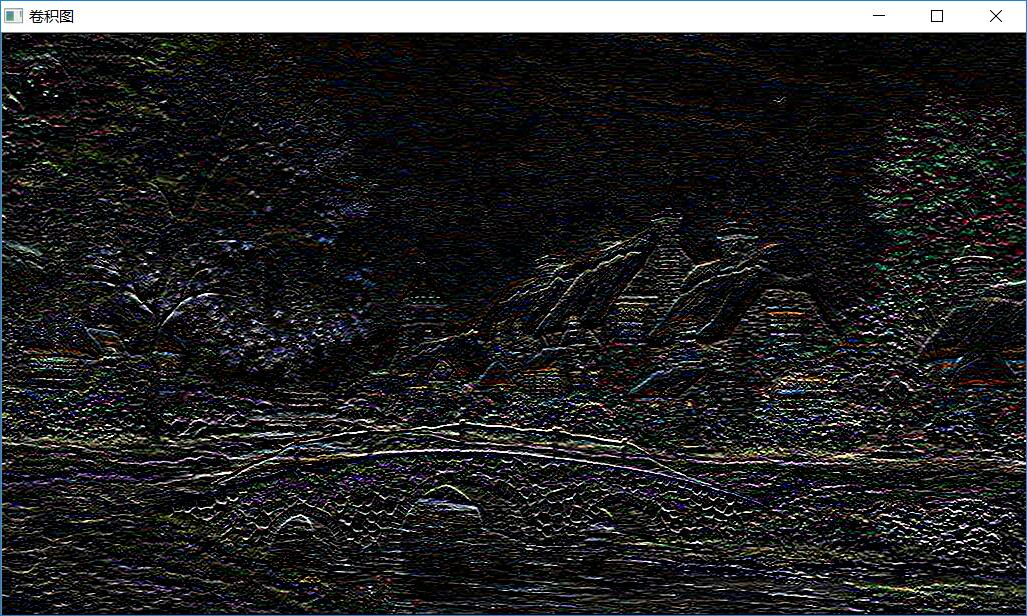## 梯度Laplacian：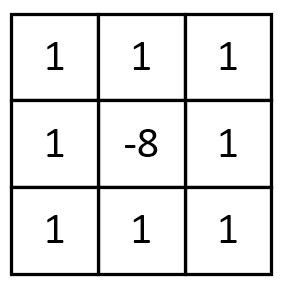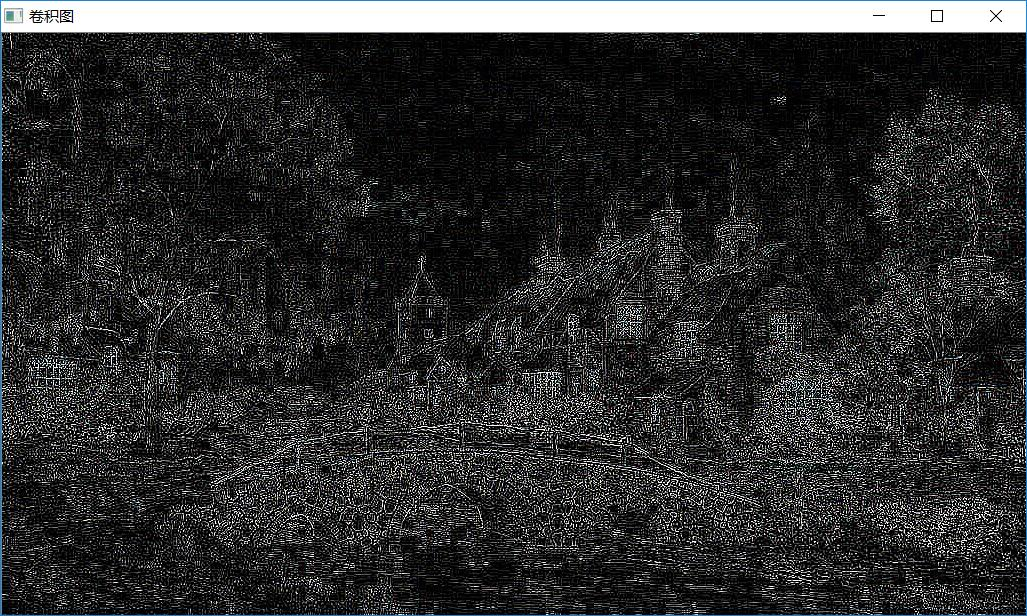Laplacian也是一种锐化方法，同时也可以做边缘检测，而且边缘检测的应用中并不局限于水平方向或垂直方向，这是Laplacian与soble的区别。下面这张图可以很好的表征出二者的区别：来源于OpenCV官方文档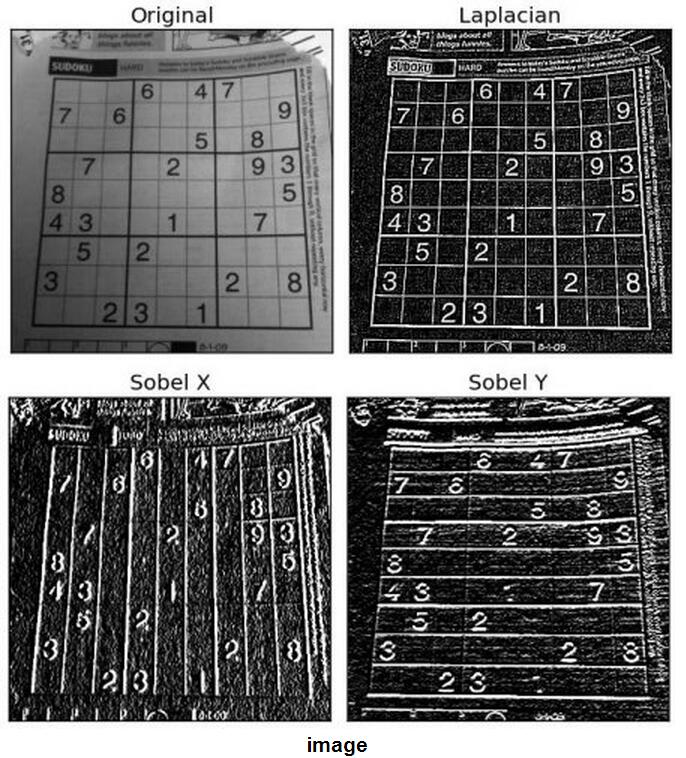# OpenCV实现

CV_EXPORTS_W void filter2D(
InputArray src,
OutputArray dst,
int ddepth,
InputArray kernel,
Point anchor=Point(-1,-1),
double delta=0,
int borderType=BORDER_DEFAULT );

#include <iostream>
#include <iostream>
#include <opencv2/core/core.hpp>
#include <opencv2/highgui/highgui.hpp>
#include <opencv2/imgproc/imgproc.hpp>
using namespace std;
using namespace  cv;

int main()
{
namedWindow("srcImage", WINDOW_AUTOSIZE);
imshow("原图", srcImage);
Mat kernel = (Mat_<double>(3,3) <<
-1, 0 ,1,
-2, 0, 2,
-1, 0, 1);
Mat dstImage;
filter2D(srcImage,dstImage,srcImage.depth(),kernel);
namedWindow("dstImage",WINDOW_AUTOSIZE);
imshow("卷积图",dstImage);
waitKey(0);
return 0;
}

# 手写卷积操作

#include <opencv2/opencv.hpp>
#include <opencv2/highgui/highgui.hpp>
#include <opencv2/core/core.hpp>

using namespace  std;
using namespace  cv;

Mat Kernel_test_3_3 = (Mat_<double>(3,3) <<
0,-1,0,
-1,5,-1,
0,-1,0);
void Convlution(Mat  InputImage,Mat  OutputImage,Mat kernel)
{
//计算卷积核的半径
int sub_x = kernel.cols/2;
int sub_y = kernel.rows/2;
//遍历图片
for (int image_y=0;image_y<InputImage.rows-2*sub_y;image_y++)
{
for(int image_x=0;image_x<InputImage.cols-2*sub_x;image_x++)
{
int pix_value = 0;
for (int kernel_y = 0;kernel_y<kernel.rows;kernel_y++)
{
for(int kernel_x = 0;kernel_x<kernel.cols;kernel_x++)
{
double  weihgt = kernel.at<double>(kernel_y,kernel_x)   ;
int value =  (int)InputImage.at<uchar>(image_y+kernel_y,image_x+kernel_x);
pix_value +=weihgt*value;
}
}
OutputImage.at<uchar>(image_y+sub_y,image_x+sub_x) = (uchar)pix_value;
//OutputImage.at<uchar>(image_y+sub_y,image_x+sub_x) = saturate_cast<uchar>((int)pix_value);
if ((int)pix_value!=(int)saturate_cast<uchar>((int)pix_value))
{
//cout<<"没有防溢出"<<(int)pix_value<<endl;
//cout<<"防溢出"<<(int)saturate_cast<uchar>((int)pix_value)<<endl;
//cout<<"没有防溢出写入了什么？"<<(int)OutputImage.at<uchar>(image_y+sub_y,image_x+sub_x)<<endl;
//cout<<endl;
}
}
}
}

int main()
{
namedWindow("srcImage", WINDOW_AUTOSIZE);
imshow("原图", srcImage);

//filter2D卷积
Mat dstImage_oprncv(srcImage.rows,srcImage.cols,CV_8UC1,Scalar(0));;
filter2D(srcImage,dstImage_oprncv,srcImage.depth(),Kernel_test_3_3);
imshow("filter2D卷积图",dstImage_oprncv);
imwrite("1.jpg",dstImage_oprncv);

//自定义卷积
Mat dstImage_mycov(srcImage.rows,srcImage.cols,CV_8UC1,Scalar(0));
Convlution(srcImage,dstImage_mycov,Kernel_test_3_3);
imshow("卷积图3",dstImage_mycov);
imwrite("2.jpg",dstImage_mycov);

waitKey(0);
return 0;

}
07-182万+07-063438
03-202万+
01-22379
10-153万+
08-314213
07-114189
01-1719万+
05-282万+
10-215万+
©️2020 CSDN 皮肤主题: Age of Ai 设计师:meimeiellie